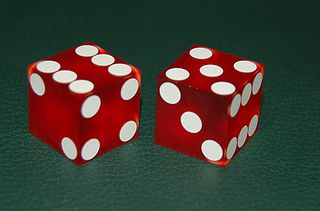Quantitative Finance

# Discrete Probability - Problem SolvingIf 2 fair six-sided dice are tossed, what is the probability that they will show the same number?

Bret and Shawn are playing a game where each turn the players roll a 20-sided fair die and then add the number they get to their score. The winner is the player with the highest score at the end of the game (there may be a tie). Before the last turn, Shawn is ahead by 10 points. The probability that Bret can come back and win the game can be expressed as $$\frac{a}{b}$$ where $$a$$ and $$b$$ are coprime numbers. What is $$a + b$$?

Details and assumptions

Both Shawn and Bret have 1 more roll on the last turn.

If there is a tie, no one won.

John has 3 unique coins such that the probability of obtaining a head when the coin is flipped is $$\frac{1}{10}$$, $$\frac{2}{10}$$ and $$\frac{3}{10}$$, respectively. If he flips each of the 3 coins once, the probability that at least 1 heads appears is $$p$$. What is the value of $$1000p$$?

John has a bag of colored billiard balls which are labeled with the numbers $$1, 2, 3, 4, 5, 6$$. He reaches into the bag, draws out a ball, records down the number and then places the ball back in the bag. He does this a total of 4 times (hence has 4 numbers). The probability that at least $$2$$ of the numbers are equal is $$\frac{a}{b}$$, where $$a$$ and $$b$$ are positive, coprime integers. What is the value of $$a + b$$?

Two six-sided dice each have the numbers 1 through 6 on their faces. Neither die is fair, but they are both weighted the same. The probability of rolling a certain number on one die is given in the table below:

$$\begin{array}{c|cccccc} \mbox{number} & 1 & 2 & 3 & 4 & 5 & 6\\ \hline \mbox{probability} & \frac{1}{6} & \frac{1}{6} & \frac{1}{9} & ? & \frac{2}{9} & ?\\ \end{array}$$

If the probability that the two dice both show the same numbers is $$\left(\frac{2}{3}\right)^4$$, we can express the probability of rolling 10 on these two dice as $$\frac{a}{b}$$ where $$a$$ and $$b$$ are coprime positive integers. What is the value of $$a + b$$?

×

Problem Loading...

Note Loading...

Set Loading...